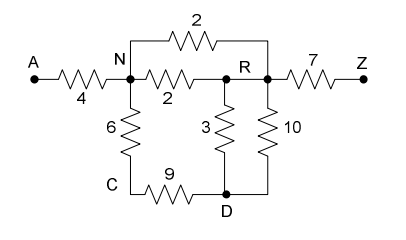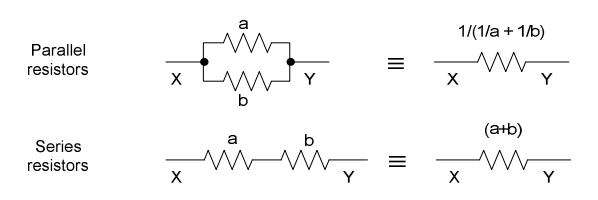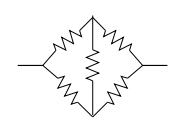시간 제한메모리 제한제출정답맞힌 사람정답 비율
1 초 128 MB51120.000%

## 문제

A series / parallel resistor circuit is shown below.The resistance value is given next to each resistor. Connection points (wires connecting two or more resistors together, are denoted by an uppercase letter. A and Z are reserved for the names of the connection points which are the endpoints of the circuit. Our goal is to calculate the equivalent resistance of the circuit (i.e., the equivalent resistance between A and Z).

Within the circuit, a resistor can be specified by a triple consisting of the connection points at either endpoint, and the resistance. Resistor 9 could be specified as either (C, D, 9) or (D, C, 9). A circuit specification is the set of all resistor specifications.

A pair of resistors is in series if one of either of their endpoints have a common connection point that is not use by any other resistor (e.g., resistor 6 and 9, are both connected to C, which is not connected to anything else). Two series resistors can be replaced by an equivalent single resistor whose resistance is the sum of the replaced resistors (15, in the previous example). A pair of resistors is in parallel if both their endpoints have common connection points (e.g., resistors 3 and 10 above, both are connected to R and D). Two parallel resistors can be replaced by an equivalent single resistor whose resistance is the inverse of the sum of the inverses of the two resistors ( (1/3 + 1/10)-1 = 2.307692, in the previous example).The equivalent resistance of a well-formed series-parallel resistor1 circuit can be determined by successively replacing a series or parallel resistor pair by the single equivalent resistor, until only one is left. If this technique fails, the circuit is not well-formed.

1A Wheatstone Bridge circuit, shown on the right, is not a well-formed series-parallel circuit.## 입력

There will be multiple circuit specifications. The first input line for each circuit specification is an integer N (N <= 1000), being the number of resistors in the circuit. This is followed by N lines, each being a resistor specification in the form: X Y r, where X and Y are uppercase characters, and r is a positive integer resistance (r < 100). The equivalent resistance is guaranteed never to be greater than 100. A line with a single 0 terminates the input.

## 출력

For each circuit, if the circuit is well-formed and reduces to a single equivalent resistance between A and Z, print the equivalent resistance of the circuit from A to Z, rounded to (and displayed to) 3 decimal places. If the circuit is not well formed, or if there is no equivalent resistance between A and Z, simply print the number -1.000. There should be no blank lines between outputs.

## 예제 입력 1

8
N R 2
D R 3
R N 2
R D 10
Z R 7
C D 9
N C 6
A N 4
2
A Z 3
Z A 10
2
P A 6
P Z 9
5
A B 1
B Z 4
A C 8
C Z 19
B C 12
0


## 예제 출력 1

11.945
2.308
15.000
-1.000